# STEP MOTOR BASICS GUIDE

A BRIEF GUIDE TO MOTOR THEORY AND DESIGN

Section 1: Motor Theory

A step motor is a constant output power transducer, where power is defined as torque multiplied by speed. This means motor torque is the inverse of motor speed. To help understand why a step motors power is independent of speed, we need to construct (figuratively) an ideal step motor.

An ideal step motor would have zero mechanical friction, its torque would be proportional to ampere-turns and its only electrical characteristic would be inductance. Ampere-turns simply mean that torque is proportional to the number of turns of wire in the motors stator multiplied by the current passing through those turns of wire.

Anytime there are turns of wire surrounding a magnetic material such as the iron in the motors stator, it will have an electrical property called inductance. Inductance describes the energy stored in a magnetic field anytime current passes through this coil of wire.

Inductance (L) has a property called inductive reactance, which for the purposes of this discussion may be thought of as a resistance proportional to frequency and therefore motor speed.

According to Ohms law, current is equal to voltage divided by resistance. In this case we substitute inductive reactance for resistance in Ohms law and conclude motor current is the inverse of motor speed.

Since torque is proportional to ampere-turns (current times the number of turns of wire in the winding), and current is the inverse of speed, torque also has to be the inverse of speed.

In an ideal step motor, as speed approaches zero, its torque would approach infinity while at infinite speed torque would be zero. Because current is proportional to torque, motor current would be infinite at zero as well.

Electrically, a real motor differs from an ideal one primarily by having a non-zero winding resistance. Also, the iron in the motor is subject to magnetic saturation, as well as having eddy current and hysteresis losses. Magnetic saturation sets a limit on current to torque proportionally while eddy current and hysteresis (iron losses) along with winding resistance (copper losses) cause motor heating.

Section 2: Speed-Torque Curve  Basics

In the previous section it was shown that motor torque varies inversely with speed. This then is the motors natural speed-torque curve. Below a certain speed, called the corner speed, current would rise above the motors rated current, ultimately to destructive levels as the motors speed is reduced further. This can be seen in Figure 1.Figure 1

To prevent this, the drive must be set to limit the motor current to its rated value. Because torque is proportional to current, motor torque is constant from zero speed to the corner speed. Above the corner speed, motor current is limited by the motors inductive reactance.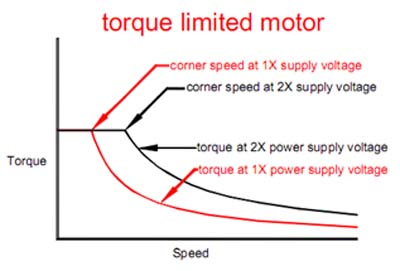Figure 2

The result now is a two-part speed-torque curve which features constant torque from zero speed until it intersects the motors natural load line, called the corner speed, beyond which the motor is in the constant power region. This is illustrated in Figure 2.

A real step motor has losses that modify the ideal speed-torque curve. The most important effect is the contribution of detent torque. Detent torque is usually specified in the motor datasheet. It is always a loss when the motor is turning and the power consumed to overcome it is proportional to speed. In other words, the faster the motor turns the greater the detent torque contributes power loss at the motors output shaft. This power loss is proportional to speed and must be subtracted from the ideal, flat output power curve past the corner speed. This now constitutes a practical speed-torque curve.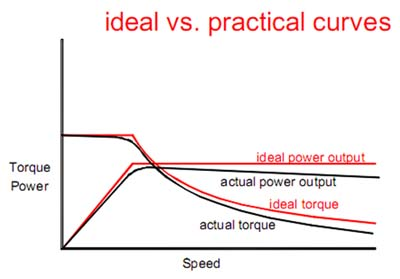Figure 3

Notice how in Figure 3 the power output decreases with speed because of the constant-torque loss due to detent torque and other losses. The same effect causes a slight decrease in torque with speed in the constant torque region as well. Finally, there is a rounding of the torque curve at the corner speed because the drive gradually transitions from being a current source to being a voltage source. The drive limits current to the motor below the corner speed and thus is a current source. Above the corner speed, the motors inductive reactance limits the current and the drive becomes a voltage source as it applies all of the power supply voltage to the motor.

Section 3: Mid-Band Instability

A step motor is highly resonant because it is a mass-spring system. The mass portion is the rotor and load inertia while the spring portion is the restoring torque of the magnetic field that drags the rotor along. Because of this, velocity lags torque by 90 degrees.

The drive is a current source in the constant torque region and adds no additional phase lag. In the constant power region however, the drive is a voltage source so it introduces an additional 90 degree phase lag. The total phase lag now is 180 degrees, which is a setup for a sustained and building motor oscillation. This oscillation is commonly called mid-band instability or mid-band resonance.

The drive remedies this instability by adding a second-order, or viscous, damping. This damping decreases the total phase lag so the motor cannot sustain oscillation, much in the same way shock absorbers damp the mass-spring suspension of a vehicle. This is illustrated in Figure 4.

The figure below shows the effect of uncompensated mid-band resonance. Though it is possible to accelerate through the resonant region, it is not possible to operate the motor continuously in the speed band. This is because the oscillation that causes the motor to stall takes from half a second to 10 seconds to build to amplitude sufficient to stall the motor.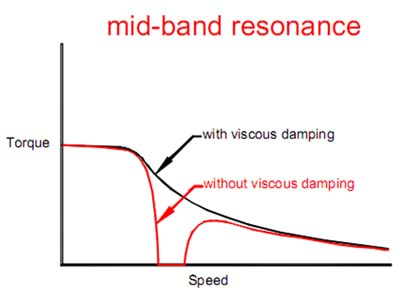Figure 4

Section 4: Motor Power Basics

The motor power output (speed times torque) is determined by the power supply voltage and the motors inductance. The motors output power is proportional to the power supply voltage divided by the square root of the motor inductance.

If one changes the power supply voltage, then a new family of speed-torque curves result. As an example, if the power supply voltage is doubled then a new curve is generated; the curve now has twice the torque at any given speed in region 2. Since power equals torque times speed, the motor now generates twice as much power as well. This is illustrated in Figure 5.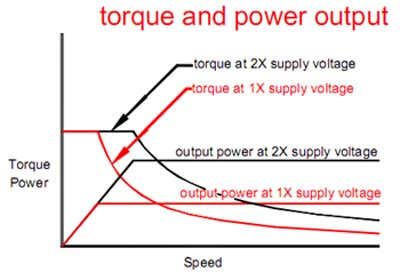Figure 5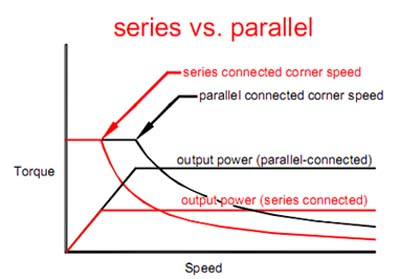Figure 6

Figure 6 shows the effect of rewiring the motor from full-winding to half-winding while keeping the same power supply voltage. A half-winding connected motor delivers twice as much power as a full- winding connection at a given power supply voltage. This is because full-winding inductance is four times higher than half-winding inductance.

Also note from Figure 5 that motor output doubles when the power supply voltage is doubled for either series or parallel-wired motors. Notice that a parallel-connected motor delivers performance identical to a series-connected motor running at twice the power supply voltage.

Figure 7 shows the effect of setting the motor current to twice the rated value. This abuses the motor because it will dissipate four times as much heat as setting the current to its proper value. The actual increase in low-speed torque is considerably less than double because of magnetic saturation of the motor iron.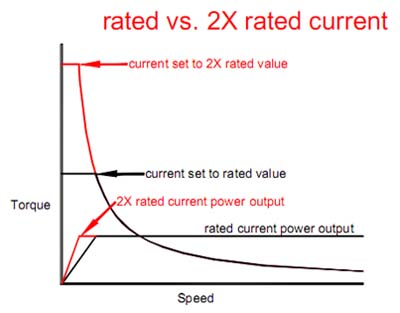Figure 7

What can be seen is there is not increase of the power output; the motor simply reaches its maximum power at a lower speed, all at the great expense of a four-fold increase in motor heating.

It is recommended the motor current always be set at the rated value also to get the best microstep smoothness. Setting the current higher degrades the linearity of the motor and causes microstep bunching and attendant low-speed vibration.

What comes with the increased motor power with increased power supply voltage is increased motor heating; this heating increases more rapidly than output power and ultimately sets the maximum output power from the motor. That is to say, the limiting factor in how much power a motor can deliver is ultimately determined by how much heat it can safely dissipate.

Section 5: Motor Connections

Step motors have four, six or eight wires; older motors may have five wires, but they will not be covered here.

Four-wire motors are the simplest to connect and offer no connection options. Simply connect one winding to the terminals labeled Phase A and Phase /A and connect the other winding to the terminals that say Phase B and Phase /B. If it is unknown which wires belong to which phase, simply use an ohmmeter and test which wires have continuity. The ones that have continuity will belong to the same phase; if the motor turns the wrong direction when connected just swap Phase A and Phase

/A. A typical four wire motor connection is illustrated in Figure 8.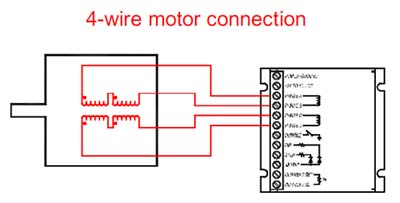Figure 8

Six-wire motors are the most common. There are two connection options: Full-winding and half- winding. A six wire motor is just like a four wire motor except there is a center tap on each of two windings, for a total of six wires. For a half-winding connection, the center tap and one of the end wires are used. This is illustrated in Figure 9.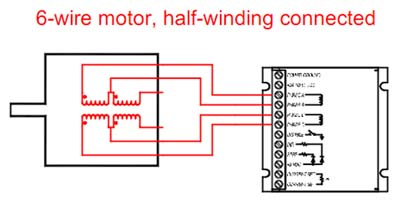Figure 9

For a full-winding connection as seen in Figure 10, the center tap is ignored and both end wires are used. The term full-winding is exactly equivalent to series connected while half-winding is virtually identical to parallel connected. The choice between the two is application dependent, which is discussed later; just remember to set the drive current to exactly half the motors rated unipolar current rating if it is wired in full-winding and set it to the unipolar current rating if wired in half-winding.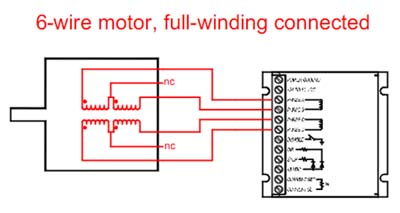Figure 10

Eight-wire motors are about 3% more efficient when parallel connected than an equivalent half- winding connected six-wire motor, but are considerably more complicated to connect. There is no advantage when comparing a series connection to a full-winding connection. As in a six-wire motor, the choice between series versus parallel connection is application dependent. Remember to set the drive current to exactly half of the motors rated parallel (as wired in Figure 11) current rating when using the series connection shown in Figure 12.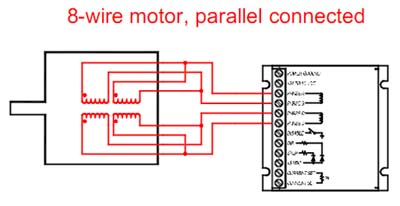Figure 11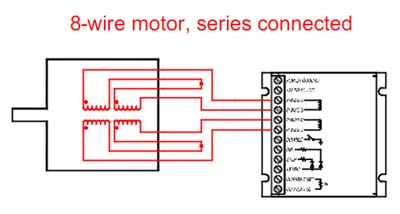Figure 12

Section 6: Power Supplies

The choice of a power supply is determined by voltage, current, and power supply type (i.e. switching versus linear, regulated versus unregulated, etc.). By far the most problematic and complicated factor is voltage, which will be discussed last.

The easiest factor in choosing a power supply is its current rating, which is based on your motor ratings. A motor control will always draw less than 2/3 of the motors rated current when it is parallel (or half-winding) connected and 1/3 of the motors rated current when it is series (or full-winding) connected. That is to say, a 6 amp per phase motor will require a 4 amp power supply when wired in parallel and a 2 amp power supply when wired in series. If multiple motors and drives are used, add the current requirements of each to arrive at the total power supply current rating.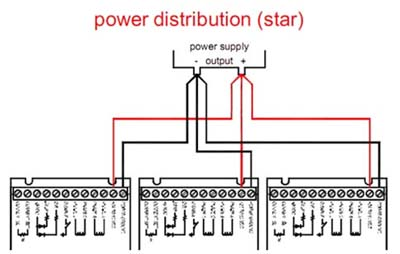Figure 13

When using multiple drives from a common power supply, use individual supply and ground wires to each drive and return them to a common point back at the power supply. This is called a star power supply distribution; never use a daisy-chain power distribution, where the supply and ground wires for the next drives are picked up from the previous one.

The voltage of your power supply is entirely dependent on the inductance rating of your motor, which we learned is translatable to the number of turns of wire in the stator. Every motor model will have a different inductance rating and will therefore have a different maximum voltage. To figure out what the maximum power supply voltage should be, use the following formula with the motors inductance in millihenries (mH) used for the L value.

32 * √L = VMAX

If you are using several different models of motors on the same power supply use the lowest inductance rating in the above formula. This will ensure that your motors will not overheat due to the voltage being too high.

Should a motor not list the inductance it will generally list the voltage rating of each winding, which will be very low. An acceptable way of determining your power supply voltage if this is the only information you have is to multiply this number by any number between 4 and 20. In Figure 14 you could use a power supply voltage anywhere from 8.8V to 44V if wired in parallel.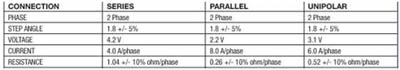Figure 14

An unregulated power supply will be sufficient and is recommended for most applications because of its simplicity. If a motor with a large inertial load decelerates quickly it will act as an alternator and send voltage back to the drive which then sends it back to the power supply. Because many regulated power supplies feature protection circuitry this may cause the power supply to fault or reset; however, if the supply is unregulated it will simply get absorbed by the filter capacitor.

To make your own power supply you must have three key components: a transformer, bridge rectifier, and filter capacitor. The transformers current rating must be sufficient to adequately run all motors that will be run from it using the above current formula. The DC output voltage will be 1.4 times the transformers AC voltage rating of the secondary. For example, a 24VAC transformer secondary will provide about 34VDC at the output of the supply. The bridge rectifiers voltage and current ratings must exceed what the supply will deliver. Finally the minimum filter capacitor size must be calculated. Use the following equation to do this:

(80,000 * I) / V = C

The result will be in microfarads for the capacitor if the value for I is amperes of current needed and V is the output voltage of the supply. When picking the capacitor, any value equal to or greater than the calculated value can be used. Be sure to use a capacitor with a voltage rating at least 20% higher than the output voltage of the power supply. A sample 68VDC 5A power supply is shown in Figure 15.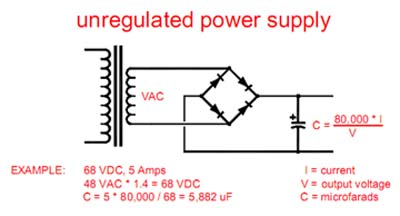Figure 15

There is a special consideration if the power supply will be at or near the maximum voltage rating of the drive. If the motor will be rapidly decelerating a large inertial load from a high speed, care has to be taken to absorb the returned energy. The energy stored in the momentum of the load must be removed during deceleration and be safely dissipated. Because of its efficiency, the drive has no means of dissipating this energy so it returns it to the power supply. In effect, instead of drawing current from the power supply, the drive becomes a source of current itself. This current may charge the power supply capacitor to destructive voltage levels.

If more than one drive is being operated from the power supply this is not a problem since the other drive(s) will absorb this current for its needs, unless it is decelerating as well. For this case or for a single drive it may be necessary to place a voltage clamp across the power supply in the form of a Zener diode. The voltage of this diode must be greater than the maximum expected power supply voltage, yet low enough to protect the drive. A good choice would be either 82 volts or 91 volts as standard values.

Section 7: Motor Heating and Power Supply Voltage

There are two major causes of motor heating: copper losses and iron losses. Copper losses are the easiest to understand; this is the heat generated by current passing through a resistance, as in the current passing through the motors winding resistance. Often this is referred to as I2R dissipation. This cause of motor heating is at a maximum when the motor is stopped and rapidly diminishes as the motor speeds up since the inductive current is inversely proportional to speed.

Eddy current and hysteresis heating are collectively called iron losses. The former induces currents in the iron of the motor while the latter is caused by the re-alignment of the magnetic domains in the iron. You can think of this as friction heating as the magnetic dipoles in the iron switch back and forth. Either way, both cause the bulk heating of the motor. Iron losses are a function of AC current and therefore the power supply voltage.

As shown earlier, motor output power is proportional to power supply voltage, doubling the voltage doubles the output power. However, iron losses outpace motor power by increasing non- linearly with increasing power supply voltage. Eventually the point is reached where the iron losses are so great that the motor cannot dissipate the heat generated. In a way this is natures way of keeping someone from getting 500HP from a NEMA 23 motor by using a 10kV power supply.

At this point it is important to introduce the concept of overdrive ration. This is the ration between the power supply voltage and the motors rated voltage. An empirically derived maximum is 25:1, meaning the power supply voltage should never exceed 25 times the motors rated voltage or 32 times the square root of motor inductance. Below is a graph of measured iron losses for a 4A, 3V motor. Notice in Figure 16 how the iron losses range from insignificant to being the major cause of heating in the motor compared to a constant 12W copper loss (4A times 3V).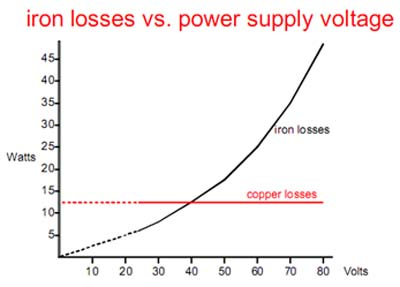Figure 16

Section 8: Accuracy and Resolution

Step motors by and large are used in open loop positioning and velocity applications. There is not feed-back transducer to set the ultimate accuracy of the system. Consequently it falls on the motor and the drives precision and behavior to determine the accuracy of the application.

Through microstepping, second order damping and precision sine/cosine current references, the drive has cured the step motor of its inherent vices to make it a candidate for precision motion control applications. Neglecting the drive, the motor still has characteristics that must be considered in regards to ultimate accuracy in any application.

A step motor is a mechanical device that is manufactured to a certain tolerance. Typically a standard motor has a tolerance of +/- 5% non accumulative error regarding the location of any given step. This means that any step on a typical 200 step per revolution motor will be within a 0.18-degree error range. Stated otherwise, the motor can accurately resolve 2000 radial locations. Coincidentally this is the resolution of a 10 microstep drive.

Any microstep resolution beyond 10, such as 125, yields no additional accuracy, only empty resolution. By analogy, a voltmeter having a 6 digit display while having 1% accuracy would have meaningful information only in the first two digits. There are two exceptions justifying higher resolutions: The step motor is being run in a closed-loop application with a high-resolution encoder or the application requires smooth operation at very low speeds (below 5 full steps per second).

Another factor affecting accuracy is motor linearity. Motor linearity refers to how the motor behaves between its ordinal step locations. Ideally a 1.8 degree per step motor should move exactly

0.18 degrees for every step pulse sent to a 10 microstep drive. In reality all step motors exhibit some non-linearity, meaning the microsteps bunch together rather than being spread evenly over the span of a full step. This has two effects: Statically the motor position is not optimum and dynamically low speed resonances occur because of the cyclic acceleration where the microsteps are spread apart and deceleration where they bunch up. Figure 17 shows a motor with excellent linearity and one with horrible linearity.

Finally, the static friction load applied to the motor affects accuracy. A stopped motor, which has 100 oz/in of holding torque, is fundamentally different than a brake that has the same holding torque.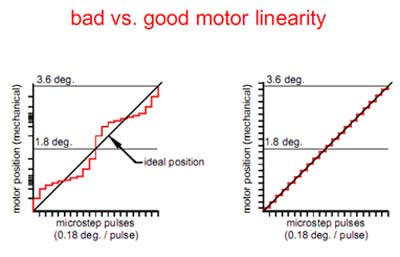Figure 17

The brake will not turn at all until its holding torque is exceeded. However a step motor only generated restoring torque if it is displaced from its rest position. Using the brake analogy, think of the output shaft being connected to the break with a torsional spring; now when applying a load, the output shaft has to be laterally displaced to apply torque to the brake.

When lateral torque sufficient enough to overcome the holding torque is applied to a step motor, the shaft will jump to the next stable location which is four full steps ahead or behind the original one, depending on the direction of the lateral torque. Peak restoring torque occurs a full step ahead or behind the original location, beyond which it weakens and reverses at the two full step position to attract the shaft to a four full step location ahead or behind the original one.

The relationship between restoring torque and shaft error angle is approximately sinusoidal as shown in Figure 18.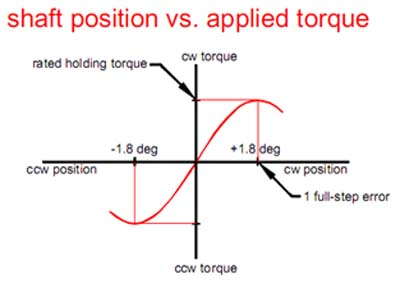Figure 18

From this one may approximate that a static torque load equal to 15 percent of the holding torque will displace the motor shaft one tenth of a full step from the origin.

Section 9: Choosing a Step Motor and Power Supply Voltage

The choice of a stepper motor and power supply voltage is entirely application dependent.

Ideally the motor should deliver sufficient at the highest speed the application requires and no more.

Any torque capability in excess of what the application requires comes at the high cost of unnecessary motor heating. Excess torque capability beyond a reasonable safety margin will never be used but will exact the penalty of an oversized power supply, drive stress and motor temperature.

Learn to distinguish the difference between torque and power; high initial torque at low speed does not mean efficient motor utilization. Usually, power is the more important measure of a motors suitability to an application. To determine this, you must bias the motors operating point through power transmission gearing to operate the motor at its maximum power; normally just past its corner frequency.

The maximum shaft power sustainable with a drive running at 80VDC and 7A is around 250W, or one third of a horsepower. This is primarily achieved with double or triple stacked NEMA 34 motors.

NEMA 23 motors are physically too small to dissipate the resultant hear and NEMA 42 motors are too big to be properly impedance matched; if their current is less than a 7A drives limit then the voltage will generally be above the maximum voltage of 80VDC and vice versa.

The detent torque on a NEMA 42 motor is significantly higher than in smaller motors and is always a loss that must be subtracted from the potential available power output of the motor. In other words, the output power of a NEMA 42 motor drops more rapidly with speed than smaller motors. A NEMA 42 motor should be used only if high torque is required at low speed and it is not practical to gear down a smaller motor.

An efficient motor, defined as the smallest motor sufficient to meet the demands of the application, will run hot. Think of the motor as having fixed power conversion efficiency: Some percentage of the input power will be converted to heat and the rest will be converted to mechanical power. To get the maximum performance from the motor, the waste heat must be just under what the motor can tolerate. Usually this motor will be biased to operate just past the corner speed as well

The place to start is to determine the load torque in oz/in, including the torque necessary to accelerate the load. The next step is to come up with the maximum speed the application has to operate at in full steps per second using the formula below. RPI is the revolutions per inch after the motor turns through the transmission, RPS is revolutions per second and PPS is the number of pulses per second from your step pulse source.

(Desired IPM * RPI) / 60 = RPS RPS * 200 = PPS

Multiply the PPS value by the number of oz/in determined previously and divide the total by 4506. The answer will be how many watts mechanical are required from the motor to meet the load from the application. When picking a motor, choose one with 40% more than the calculated power. Below is an example of the equation completed for a load requiring 450 oz/in with a 3 TPI leadscrew and a desired IPM of 300.

(300 * 3) / 60 = 15

15 * 200 = 3000

(3000 * 450) / 4506 = 299 oz/in

299 * 1.4 = 419 oz/in

As you can see, you will want to use a motor with a rating of 419 oz/in for this application.